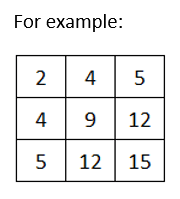# Input numbers in 2d array and check symmetric matrix or not in Java

###### Two Dimensional Array - Question 10

In this question, we will see how to input numbers in a 3X3 integer matrix (2d array) and check whether it is a symmetric matrix or not in Java programming. To know more about two dimensional array click on the two dimensional array lesson.

Q10) Write a program in Java to input numbers in a 3X3 integer matrix (2d array) and check whether it is a symmetric matrix or not. Symmetric matrix is such a matrix whose row elements are exactly same as column elements.#### Program

``````import java.util.Scanner;

public class Q10
{
public static void main(String args[])
{
int a[][]=new int, r,c,x=0;
Scanner sc=new Scanner(System.in);

System.out.println("Enter 9 numbers");
for(r=0; r<3; r++)
{
for(c=0; c<3; c++)
{
a[r][c]=sc.nextInt();
}
}

for(r=0; r<3; r++)
{
for(c=0; c<3; c++)
{
System.out.print(a[r][c]+" ");
if(a[r][c]==a[c][r])
{
x++;
}
}
System.out.println();
}

if(x==9)
{
System.out.println("Symmetric Matrix");
}
else
{
System.out.println("Not Symmetric Matrix");
}
}
}``````

#### Output

```Enter 9 numbers
2
4
5
4
9
12
5
12
15
2 4 5
4 9 12
5 12 15
Symmetric Matrix```